Open in App
Not now

# GUI Billing System and Menu Card Using Python

• Difficulty Level : Hard
• Last Updated : 25 May, 2022

So imagine that we’re starting a new restaurant or being appointed as one of the employees in a restaurant company, and we find out that there’s no fast method of doing the billing of customers, and it usually takes times for us to calculate the amount that a customer has to pay. This can be really annoying and time taking thing for us as well as for customers. So now what to do? Here’s when python comes to the rescue and since we know it, it will be just a few seconds of work.

So, in this article, we’re going to build a GUI billing system and a menu card with the help of python’s module Tkinter.

Step 1: Importing the tkinter package

`from tkinter import *`

Here’s only one file we have to install in this project which will work as the background image of our GUI Billing System or we can choose any other image. After downloading make sure that the python program which we are creating and these assets are in the same folder.

Image Used: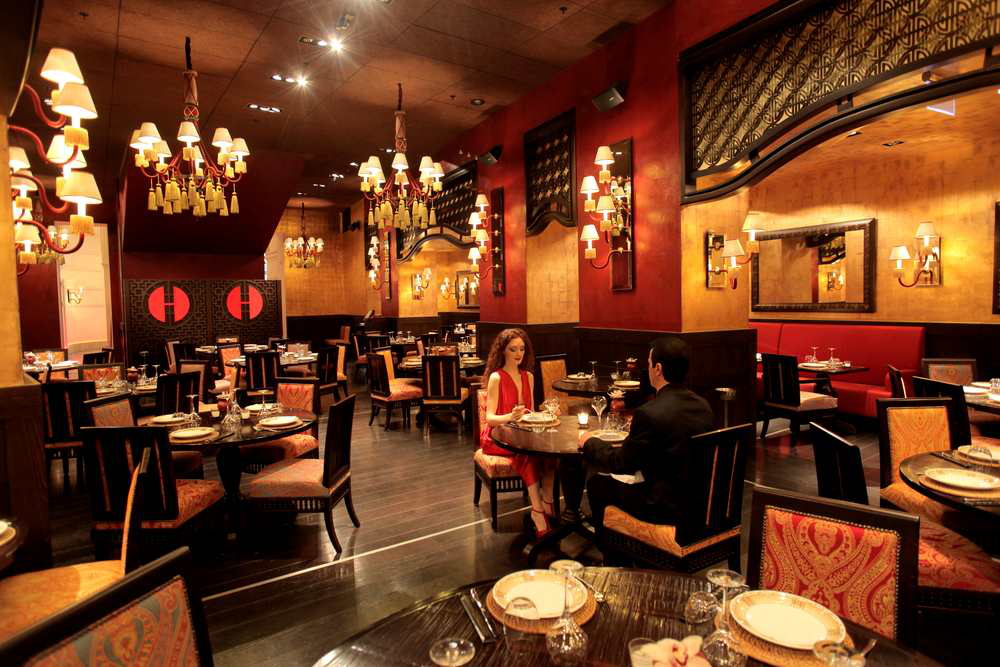Step 3: Making tkinter window and setting the background

Now we make the tkinter window and set the background for the GUI

## Python3

 `# import tkinter module ` `from` `tkinter ``import` `*` ` `  `# make a window ` `window ``=` `Tk() ` ` `  `# specify it's size ` `window.geometry(``"700x600"``) ` ` `  `# take a image for background ` `bg ``=` `PhotoImage(``file``=``'bg.png'``) ` ` `  `# label it in the background ` `label17 ``=` `Label(window, image``=``bg) ` ` `  `# position the image as well ` `label17.place(x``=``0``, y``=``0``) ` ` `  `# closing the main loop ` `window.mainloop() `

Output: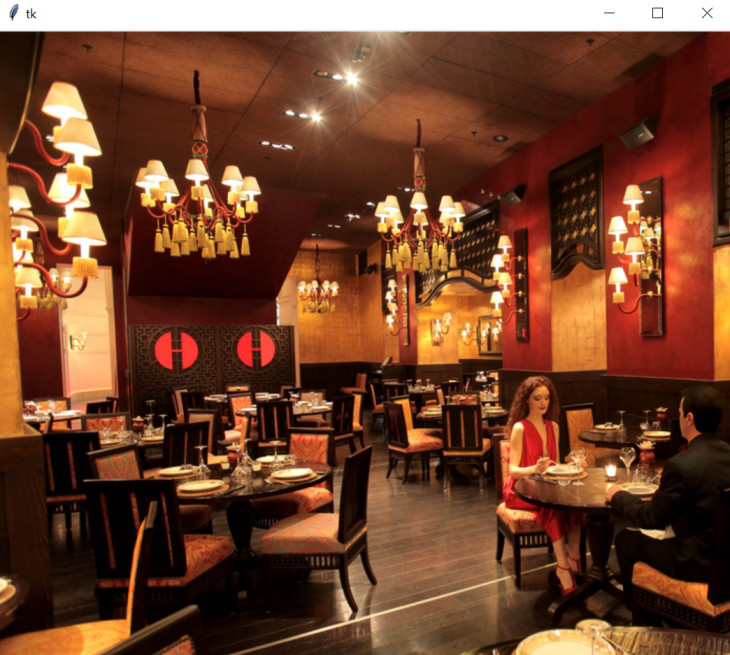Now we will add the title and menu card for the GUI billing system with help of “Label()” function. Tkinter Label is a widget that is used to implement display boxes where we can place text or images. The text displayed by this widget can be changed by the developer at any time you want. It is also used to perform tasks such as underlining the part of the text and span the text across multiple lines. It is important to note that a label can use only one font at a time to display text. To use a label, we just have to specify what to display in it (this can be text, a bitmap, or an image).

Syntax: w = Label ( master, option, … )

Parameters:

• master: This represents the parent window
• options: These options can be used as key-value pairs separated by commas

## Python3

 `# main title ` `label8 ``=` `Label(window, text``=``"Saransh Restaurant"``, ` `               ``font``=``"times 28 bold"``) ` `label8.place(x``=``350``, y``=``20``, anchor``=``"center"``) ` ` `  `# Menu Card ` `label1 ``=` `Label(window, text``=``"Menu"``, ` `               ``font``=``"times 28 bold"``) ` `label1.place(x``=``520``, y``=``70``) ` ` `  `label2 ``=` `Label(window, text``=``"Aloo Paratha Rs 30"``, ` `               ``font``=``"times 18"``) ` `label2.place(x``=``450``, y``=``120``) ` ` `  `label3 ``=` `Label(window, text``=``"Samosa  Rs 5"``, ` `               ``font``=``"times 18"``) ` `label3.place(x``=``450``, y``=``150``) ` ` `  `label4 ``=` `Label(window, text``=``"Pizza   Rs 150"``, ` `               ``font``=``"times 18"``) ` `label4.place(x``=``450``, y``=``180``) ` ` `  `label5 ``=` `Label(window, text``=``"Chilli Potato  Rs 50"``, ` `               ``font``=``"times 18"``) ` `label5.place(x``=``450``, y``=``210``) ` ` `  `label6 ``=` `Label(window, text``=``"Chowmein   Rs 70"``, ` `               ``font``=``"times 18"``) ` `label6.place(x``=``450``, y``=``240``) ` ` `  `label7 ``=` `Label(window, text``=``"Gulab Jamun  Rs 35"``, ` `               ``font``=``"times 18"``) ` `label7.place(x``=``450``, y``=``270``) ` ` `  `# closing the main loop ` `window.mainloop() `

Output: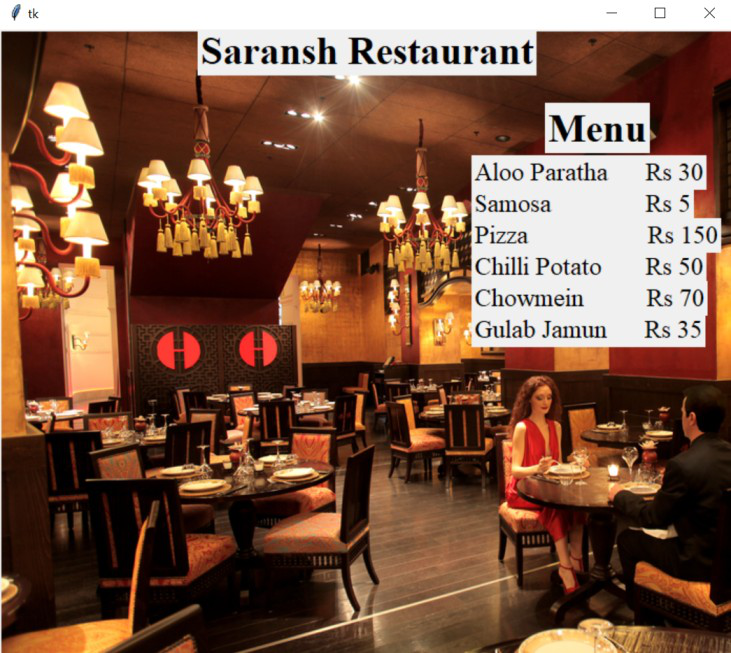Step 5: Adding the billing section

Now we will add the billing section by using the same label widget and the entry widget. The Entry Widget is a Tkinter Widget used to Enter or display a single line of text. Also, Label.place(x,y) indicates the position of the label in the tkinter window.

Syntax: entry = tk.Entry(parent, options)

Parameters:

• parent: This represents the parent window
• options: These options can be used as key-value pairs separated by commas

## Python3

 `#------billing section--------- ` `label9``=``Label(window,text``=``"Select the items"``, ` `             ``font``=``"times 18"``) ` `label9.place(x``=``115``,y``=``70``) ` ` `  `label10``=``Label(window,text``=``"Aloo Paratha"``, ` `              ``font``=``"times 18"``) ` `label10.place(x``=``20``,y``=``120``) ` ` `  `e1``=``Entry(window) ` `e1.place(x``=``20``,y``=``150``) ` ` `  `label11``=``Label(window,text``=``"Samosa"``, ` `              ``font``=``"times 18"``) ` `label11.place(x``=``20``,y``=``200``) ` ` `  `e2``=``Entry(window) ` `e2.place(x``=``20``,y``=``230``) ` ` `  `label12``=``Label(window,text``=``"Pizza"``, ` `              ``font``=``"times 18"``) ` `label12.place(x``=``20``,y``=``280``) ` ` `  `e3``=``Entry(window) ` `e3.place(x``=``20``,y``=``310``) ` ` `  `label13``=``Label(window,text``=``"Chilli Potato"``, ` `              ``font``=``"times 18"``) ` `label13.place(x``=``20``,y``=``360``) ` ` `  `e4``=``Entry(window) ` `e4.place(x``=``20``,y``=``390``) ` ` `  `label14``=``Label(window,text``=``"Chowmein"``, ` `              ``font``=``"times 18"``) ` `label14.place(x``=``250``,y``=``120``) ` ` `  `e5``=``Entry(window) ` `e5.place(x``=``250``,y``=``150``) ` ` `  `label15``=``Label(window,text``=``"Gulab Jamun"``, ` `              ``font``=``"times 18"``) ` `label15.place(x``=``250``,y``=``200``) ` ` `  `e6``=``Entry(window) ` `e6.place(x``=``250``,y``=``230``) ` ` `  `# closing the main loop ` `window.mainloop()  `

Output: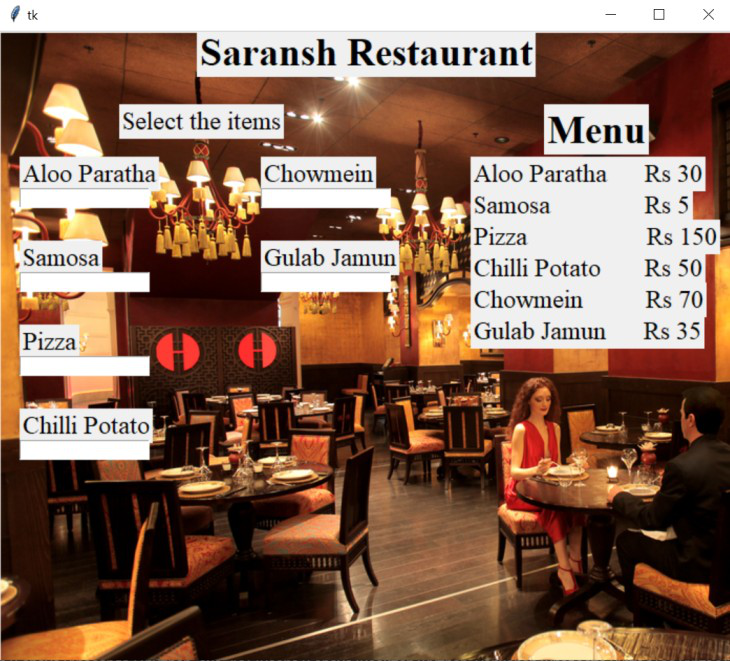Step 6: Calculating the bill and refreshing the window

After that, we have to add the calculate function which will get executed every second. In the calculate function we have to do simple math where if e.get() returns an empty string then it means that there’s no quantity selected for that particular food, else if there’s any value present in the e.get() then since it is of string type we convert it to int type and multiply this quantity of food with the price of that food. The food variable along with its quantity and price is kept in a dictionary. We look for every key in the dictionary and accordingly increment our ‘total’  variable. After that, we make another label where we use the total variable’s value to display the total amount of foods ordered. Then we made a command that after every 1000 milliseconds we refresh the window to again calculate the total amount of foods ordered which will update our GUI. Also, the total amount label gets updated by destroying the previous one and updating it with a new one every second.

## Python3

 `# function to calculate the ` `# price of all the orders ` ` `  ` `  `def` `calculate(): ` `   `  `   ``# dic for storing the food quantity and price ` `    ``dic ``=` `{``'aloo_partha'``: [e1, ``30``], ` `           ``'samosa'``: [e2, ``5``], ` `           ``'pizza'``: [e3, ``150``], ` `           ``'chilli_potato'``: [e4, ``50``], ` `           ``'chowmein'``: [e5, ``70``], ` `           ``'gulab_jamun'``: [e6, ``35``]} ` `    ``total ``=` `0` `     `  `    ``for` `key, val ``in` `dic.items(): ` `        ``if` `val[``0``].get() !``=` `"": ` `            ``total ``+``=` `int``(val[``0``].get())``*``val[``1``] ` `    ``label16 ``=` `Label(window, ` `                    ``text``=``"Your Total Bill is - "``+``str``(total), ` `                    ``font``=``"times 18"``) ` ` `  `    ``# position ` `    ``label16.place(x``=``20``, y``=``490``) ` ` `  `    ``# it will update the label with a new one ` `    ``label16.after(``1000``, label16.destroy) ` ` `  `    ``# refreshing the window ` `    ``window.after(``1000``, calculate) ` ` `  ` `  `# execute calculate function after 1 second ` `window.after(``1000``, calculate) ` `window.mainloop() `

Output: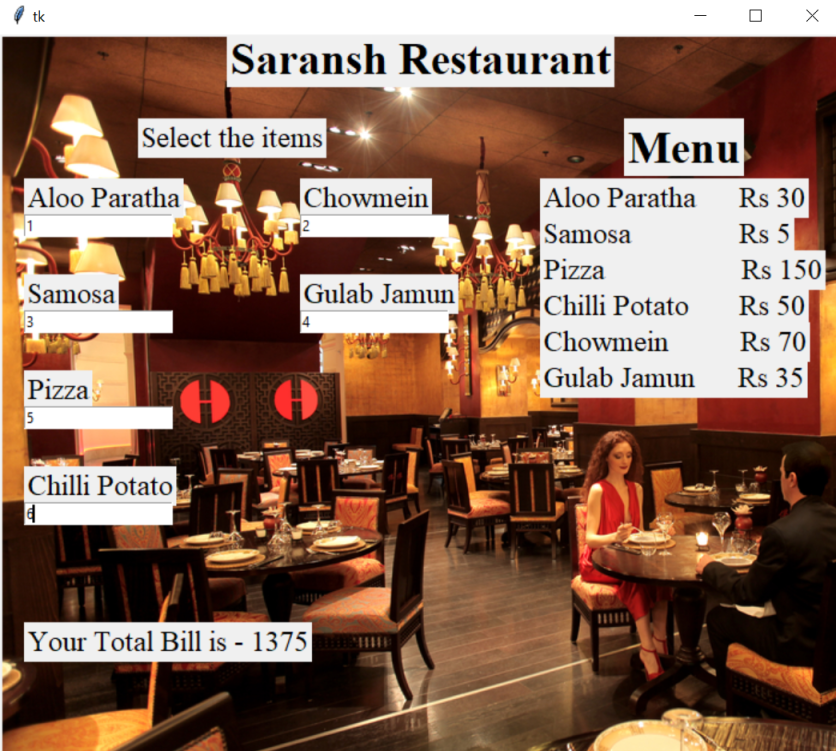Below is the full implementation:

## Python3

 `# import tkinter module ` `from` `tkinter ``import` `*` ` `  `# make a window ` `window ``=` `Tk() ` ` `  `# specify it's size ` `window.geometry(``"700x600"``) ` ` `  `# take a image for background ` `bg ``=` `PhotoImage(``file``=``'bg.png'``) ` ` `  `# label it in the background ` `label17 ``=` `Label(window, image``=``bg) ` ` `  `# position the image as well ` `label17.place(x``=``0``, y``=``0``) ` ` `  ` `  `# function to calculate the ` `# price of all the orders ` `def` `calculate(): ` ` `  `        ``# dic for storing the ` `    ``# food quantity and price ` `    ``dic ``=` `{``'aloo_partha'``: [e1, ``30``], ` `           ``'samosa'``: [e2, ``5``], ` `           ``'pizza'``: [e3, ``150``], ` `           ``'chilli_potato'``: [e4, ``50``], ` `           ``'chowmein'``: [e5, ``70``], ` `           ``'gulab_jamun'``: [e6, ``35``]} ` `    ``total ``=` `0` `    ``for` `key, val ``in` `dic.items(): ` `        ``if` `val[``0``].get() !``=` `"": ` `            ``total ``+``=` `int``(val[``0``].get())``*``val[``1``] ` ` `  `    ``label16 ``=` `Label(window, ` `                    ``text``=``"Your Total Bill is - "``+``str``(total), ` `                    ``font``=``"times 18"``) ` ` `  `    ``# position it ` `    ``label16.place(x``=``20``, y``=``490``) ` `    ``label16.after(``1000``, label16.destroy) ` `    ``window.after(``1000``, calculate) ` ` `  ` `  `label8 ``=` `Label(window, ` `               ``text``=``"Saransh Restaurant"``, ` `               ``font``=``"times 28 bold"``) ` `label8.place(x``=``350``, y``=``20``, anchor``=``"center"``) ` ` `  ` `  `label1 ``=` `Label(window, ` `               ``text``=``"Menu"``, ` `               ``font``=``"times 28 bold"``) ` ` `  `label1.place(x``=``520``, y``=``70``) ` ` `  `label2 ``=` `Label(window, text``=``"Aloo Paratha  \ ` `Rs ``30``", font="``times ``18``") ` ` `  `label2.place(x``=``450``, y``=``120``) ` ` `  `label3 ``=` `Label(window, text``=``"Samosa    \ ` `Rs ``5``", font="``times ``18``") ` ` `  `label3.place(x``=``450``, y``=``150``) ` ` `  `label4 ``=` `Label(window, text``=``"Pizza        \ ` `Rs ``150``", font="``times ``18``") ` `label4.place(x``=``450``, y``=``180``) ` ` `  `label5 ``=` `Label(window, text``=``"Chilli Potato  \ ` `Rs ``50``", font="``times ``18``") ` ` `  `label5.place(x``=``450``, y``=``210``) ` ` `  `label6 ``=` `Label(window, text``=``"Chowmein   \ ` `Rs ``70``", font="``times ``18``") ` ` `  `label6.place(x``=``450``, y``=``240``) ` ` `  `label7 ``=` `Label(window, text``=``"Gulab Jamun  \ ` `Rs ``35``", font="``times ``18``") ` ` `  `label7.place(x``=``450``, y``=``270``) ` ` `  `# billing section ` `label9 ``=` `Label(window, text``=``"Select the items"``, ` `               ``font``=``"times 18"``) ` `label9.place(x``=``115``, y``=``70``) ` ` `  `label10 ``=` `Label(window, ` `                ``text``=``"Aloo Paratha"``, ` `                ``font``=``"times 18"``) ` `label10.place(x``=``20``, y``=``120``) ` ` `  `e1 ``=` `Entry(window) ` `e1.place(x``=``20``, y``=``150``) ` ` `  `label11 ``=` `Label(window, text``=``"Samosa"``, ` `                ``font``=``"times 18"``) ` `label11.place(x``=``20``, y``=``200``) ` ` `  `e2 ``=` `Entry(window) ` `e2.place(x``=``20``, y``=``230``) ` ` `  `label12 ``=` `Label(window, text``=``"Pizza"``, ` `                ``font``=``"times 18"``) ` `label12.place(x``=``20``, y``=``280``) ` ` `  `e3 ``=` `Entry(window) ` `e3.place(x``=``20``, y``=``310``) ` ` `  `label13 ``=` `Label(window, ` `                ``text``=``"Chilli Potato"``, ` `                ``font``=``"times 18"``) ` `label13.place(x``=``20``, y``=``360``) ` ` `  `e4 ``=` `Entry(window) ` `e4.place(x``=``20``, y``=``390``) ` ` `  `label14 ``=` `Label(window, ` `                ``text``=``"Chowmein"``, ` `                ``font``=``"times 18"``) ` `label14.place(x``=``250``, y``=``120``) ` ` `  `e5 ``=` `Entry(window) ` `e5.place(x``=``250``, y``=``150``) ` ` `  `label15 ``=` `Label(window, ` `                ``text``=``"Gulab Jamun"``, ` `                ``font``=``"times 18"``) ` ` `  `label15.place(x``=``250``, y``=``200``) ` ` `  `e6 ``=` `Entry(window) ` `e6.place(x``=``250``, y``=``230``) ` ` `  `# execute calculate function after 1 second ` `window.after(``1000``, calculate) ` ` `  `# closing the main loop ` `window.mainloop() `

Output: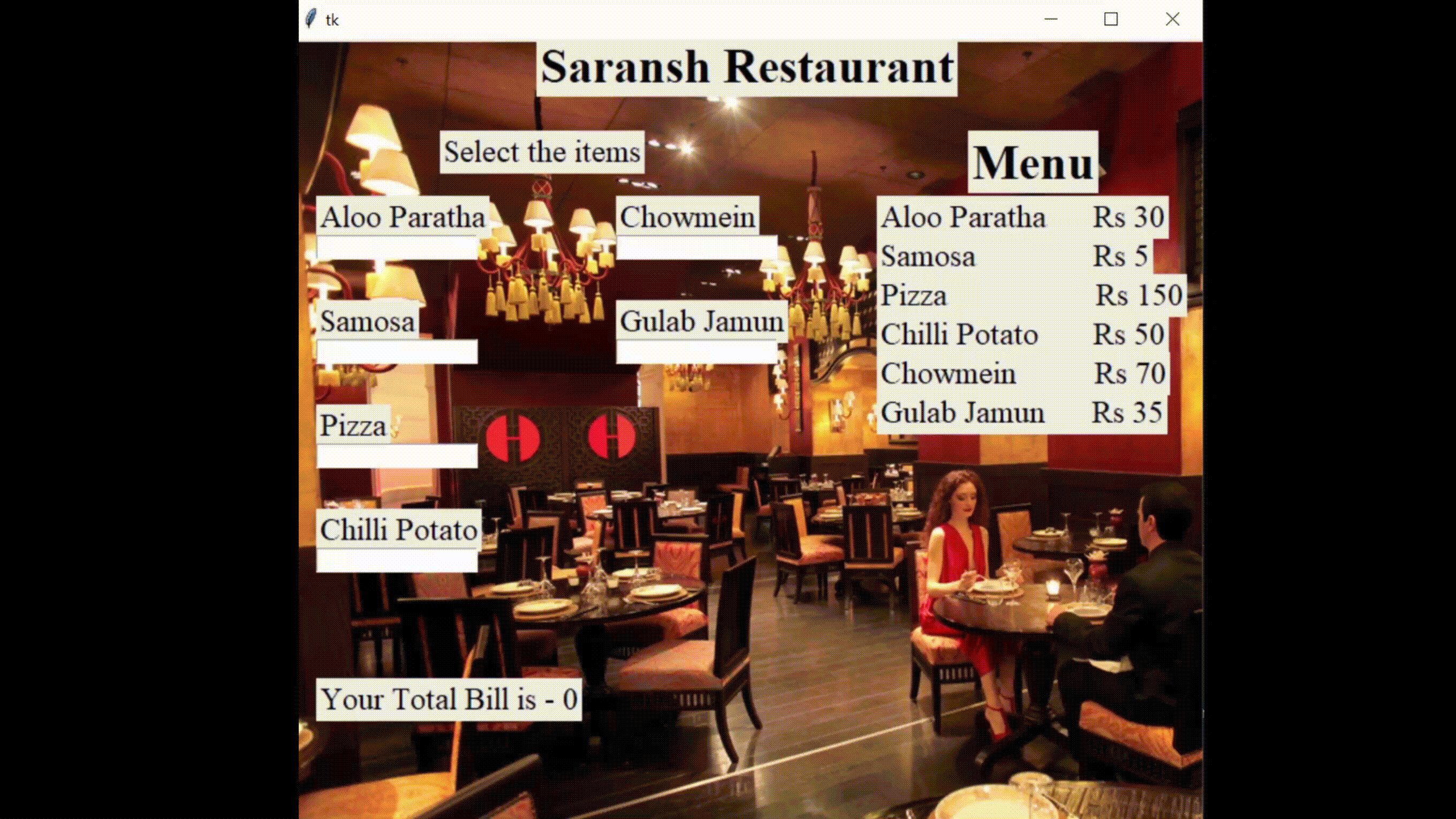My Personal Notes arrow_drop_up
Related Articles